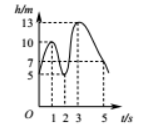$\text{A.}$ $5 \mathrm{~m}$ $\text{B.}$ $7 \mathrm{~m}$ $\text{C.}$ $10 \mathrm{~m}$ $\text{D.}$ $13 \mathrm{~m}$①点击 首页查看更多试卷和试题 , 点击查看 本题所在试卷